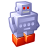## how to use Pressure Measurement Characteristic to calculate Pressure

cancel
Showing results for
Did you mean:

## how to use Pressure Measurement Characteristic to calculate Pressure

335 ViewsContributor I

hi, I'm working in a project with TPMS. using the example program i get de array gu16UUAA, looking at the formula i dont know how to calculate de pressure using that table. please any guide will be appreciated

Labels (1)
• ### Pressure Sensors

Tags (4)
105 ViewsNXP Employee

Hi Javier,

You only need to substitute the values in this transfer function.

P = ΔP1500 x PCODE + (100 – ΔP1500)

Example:

ΔP1500 = 2.750 kPa/count (Should be in the datasheet on pressure measurement characteristics)

PCODE = 0x20 = 32

P = 2.750 kpa/count x 32 count + (100 - 2.750 kPa/count)

P = 88 kPa + 97.25 kpa

P = 185.25 kPa

You can also refer to the attachment for more detail in the correspondence between compensated data in common units.

Regards,

Darío